# Wiener field

A generalization of the notion of Wiener process for the case of multivariate time. This generalization can be performed in two ways.

##-parameter Wiener field (Brownian motion).

Letbe a Gaussian separable real-valued field on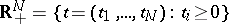with zero mean and covariance function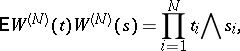where. Such a field can be regarded as the distribution function of a white noise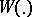on, which is a random function on bounded Borel sets insuch that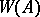has a normal distribution with zero mean and covariance function[a11]. Here,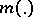denotes the Lebesgue measure on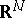. The following equality holds: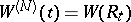, whereis a parallelepiped in.

The random field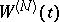was introduced by T. Kitagava [a14] in connection with its applications to statistical problems. N.N. Chentsov proved the almost sure continuity of the sample functions of[a3]. For any fixedtime variables,is a one-parameter Wiener process as a function of the free time variable. Some properties ofare similar to the corresponding properties of the Wiener process: the sample functions ofalmost surely satisfy Hölder's stochastic condition with exponent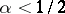[a2]; various forms of the law of the iterated logarithm hold true ([a5], [a18], [a20]). An exact formula forexists only for the Wiener process. For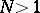, only lower and upper bounds (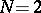, [a12]) and some asymptotic formulas for[a21] have been derived so far (1998). The level sets ofhave an extremely complicated geometric and topological structure ([a8], [a9], [a10], [a13]). R.J. Adler [a1] showed that the Hausdorff dimension of these sets equals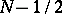.

The Wiener process is a Markov process: conditional of the present value, the past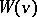() and the future(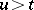) are independent. For the multivariate case there are several definitions of the Markovian property. Let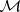denote a family of Jordan surfaces in. Each such surface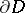dividesinto two parts:, the interior of, or the "past" , and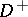, the exterior of, or the "future" . A random fieldis said to be Markovian with respect to the familyif for arbitraryfromand arbitrary,, the random variables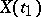andare conditionally independent given[a24]. A Wiener fieldis a Markovian field with respect to the familyconsisting of all finite unions of rectangles whose sides are parallel to the coordinate axes ([a22], [a23]). For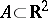, its sharp field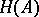and germ fieldare defined, respectively, by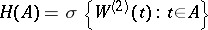and, whereis an-neighbourhood of. A Wiener sheetis germ Markovian, i.e. for every bounded subset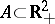, the fieldsand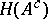are conditionally independent given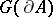([a6], [a7], [a22]).

Among the objects closely related toare the Wiener pillow and the Wiener bridge. These are Gaussian random fields (cf. also Random field) on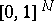with zero mean and covariance functions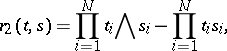respectively.

## Lévy-parameter Brownian motion.

This is a Gaussian random field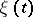onwith zero mean and covariance function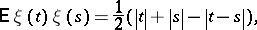where[a15]. When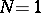,becomes a Wiener process. The random variables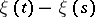clearly form a Wiener process ifmoves along some semi-straight line with terminal point.has the following representation in terms of white noise: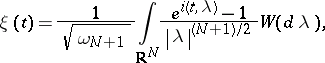where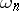is the surface area of the-dimensional unit sphere [a17]. H.P. McKean Jr. [a16] has shown thatis germ Markovian with respect to closed bounded subsets in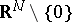for each odd, whereas for each eventhe Markovian property does not hold.